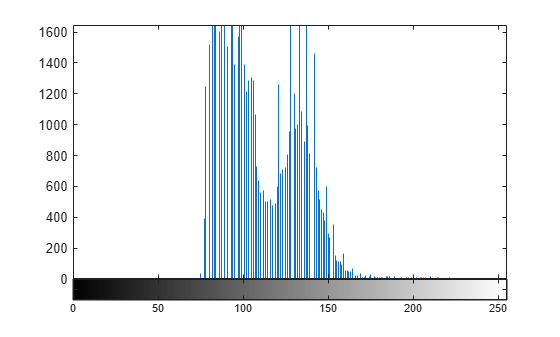# imhist

Histogram of image data

## Syntax

``````[counts,binLocations] = imhist(I)``````
``[counts,binLocations] = imhist(I,n)``
``[counts,binLocations] = imhist(X,map)``
``imhist(___)``

## Description

``````[counts,binLocations] = imhist(I)``` calculates the histogram for the grayscale image `I`. The `imhist` function returns the histogram counts in `counts` and the bin locations in `binLocations`. The number of bins in the histogram is determined by the image type.You optionally can compute the histogram counts and bin locations using a GPU (requires Parallel Computing Toolbox™).```
````[counts,binLocations] = imhist(I,n)` specifies the number of bins, `n`, used to calculate the histogram.```
````[counts,binLocations] = imhist(X,map)` calculates the histogram for the indexed image `X` with colormap `map`. The histogram has one bin for each entry in the colormap.This syntax is not supported on a GPU.```

example

````imhist(___)` displays a plot of the histogram. If the input image is an indexed image, then the histogram shows the distribution of pixel values above a colorbar of the colormap `map`.If you use this syntax when `I` is a `gpuArray`, then no plot is displayed. `imhist` returns the histogram counts in `ans` and does not return the histogram bin locations.```

## Examples

collapse all

Read a grayscale image into the workspace.

`I = imread('pout.tif');`

Display a histogram of the image. Since `I` is grayscale, by default the histogram will have 256 bins.

`imhist(I)``load mristack`

Display the histogram of the data. Since the image is grayscale, `imhist` uses 256 bins by default.

`imhist(mristack)`## Input Arguments

collapse all

Grayscale image, specified as a numeric array of any dimension.

Example: `I = imread('cameraman.tif');`

Data Types: `single` | `double` | `int8` | `int16` | `int32` | `uint8` | `uint16` | `uint32` | `logical`

Number of bins, specified as a positive integer. If `I` is a grayscale image, then `imhist` uses a default value of 256 bins. If `I` is a binary image, then `imhist` uses two bins.

Example: `[counts,x] = imhist(I,50);`

Data Types: `single` | `double` | `int8` | `int16` | `int32` | `int64` | `uint8` | `uint16` | `uint32` | `uint64`

Indexed image, specified as a numeric array of any dimension.

Example: `[X,map] = imread('trees.tif');`

Data Types: `single` | `double` | `uint8` | `uint16` | `logical`

Colormap associated with indexed image `X`, specified as a c-by-3 numeric matrix with values in the range [0, 1]. Each row is a three-element RGB triplet that specifies the red, green, and blue components of a single color of the colormap.The colormap must be at least as long as the largest index in `X`.

Example: `[X,map] = imread('trees.tif');`

Data Types: `single` | `double` | `int8` | `int16` | `int32` | `uint8` | `uint16` | `uint32` | `logical`

## Output Arguments

collapse all

Histogram counts, returned as a numeric array. If the histogram is computed for an indexed image, `X`, then the length of `counts` is the same as the length of the colormap, `map`.

Bin locations, returned as a numeric array.

## Tips

• For grayscale images, the `n` bins of the histogram are each half-open intervals of width A/(n−1). In particular, the pth bin is the half-open interval

`$\frac{A\left(p-1.5\right)}{\left(n-1\right)}-B\le x<\frac{A\left(p-0.5\right)}{\left(n-1\right)}-B,$`

where x is the intensity value. The scale factor A and offset B depend on the type of the image class as follows:

doublesingleint8int16int32uint8uint16uint32logical
A112556553542949672952556553542949672951
B001283276821474836480000

• To display the histogram from `counts` and `binLocations`, use the command `stem(binLocations,counts)`.